# Coding Challenge #2: It's sorting time!

## Coding Challenge #2

Welcome back, to the second coding challenge! I got positive feedback from the first one, so I’m excited to continue this. I decided to make this one a little bit easier, since last one was a little bit intimidating!

As usual, same requirements:

• Has to be written in Lua 5.1 (the version roblox uses)
• The program has to be compatible with Roblox Studio, meaning you can’t use features vanilla Lua has but rbxlua disabled
• New rule: you can’t use httpservice to cheat the challenge

Today’s challenge is gonna be very straight forward, implementing a quick sort. In computer science, students are introduced to sorting algorithms as head start. Many of them exist, but quick sort is the most popular among them, mainly because it’s the most efficient (average time complexity of `O(nlogn)`, if you want more info search Big O Notation as a key word). In fact, `table.sort()` uses quick sort to sort the inputted table.

A sorting algorithm is basically an algorithm that takes a disordered table of number values, and sorts it to return an ordered version of the table (from lesser to greater).

You are free to do whatever you want, the end product has to be a function `quicksort()`, that takes a table as input, and returns an ordered table.

I am going to explain to you how this algorithm works.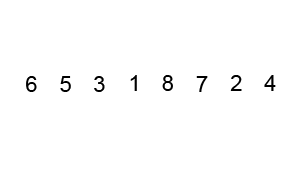(observe this gif to see how it works)

First of all, we choose a pivot. In the example below, I chose `3`, you can actually choose any member of the table as a pivot, even the first and last elements.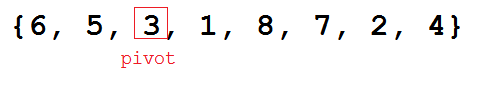The table is now, seperated to two parts, the part on the left to the pivot, and the part to the right of the pivot. Essentially, our goal is to make all the members on the right part greater than the pivot, and all the member on the left part less than the pivot.

We will choose two `pointers`, the first one will be the first element of the list, the other will be the last, it would be fine if the pointer was the pivot itself. The first pointer, the one at the start, will be going forward, and each time it finds an element greater than the pivot it stops and wait. The second pointer, the one at the end, will be going backwards, and each time it finds an element greater than the pivot it stops and wait. If both pointers stopped, they swap the elements they refer to. If one of the pointers was holding the pivot, it’s fine, it can be swapped as well.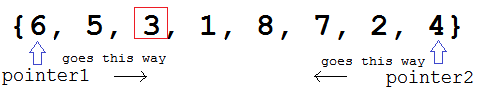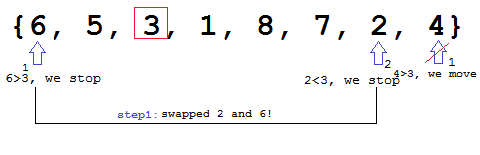And they carry on, until they hit each other and point to the same element, if that happens, we freeze the pivot, meaning it’s in its correct position, and will no longer be touched.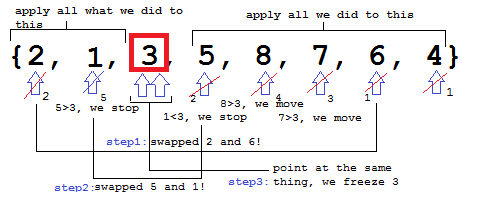After it’s frozen, you’ll notice that the array is split to two parts, what we’ll do is, apply a quicksort to both of them! Choosing a pivot again, two pointers, moving and swapping, until they hit each other. And if one of the parts was also split to two parts, we’ll implement another quicksort on both the parts, again and again and again, until we’re done!

I think you noticed, that we’re doing it recursively (recursion). And we carry on, until all elements are frozen! I hope this helped!

Since this is such a common task, the internet is FILLED with resources about this, so finding more information will be super easy, a good way to improve your searching skills! You can also find sample codes of this sorting algorithm, which can help you a lot. Which is why I think this challenge is easy! You can’t get stuck.

Anywho, this is it again! I hope you have fun with this one! You can find a list of all the challenges here or a github version here.Answer is here! Don't spoil yourself!

PLOT TWIST: this challenge will not have a well explained solution, I just think the internet has too many code samples, well explained ones, that it’s useless to do one on my own. Writing commented code is easy.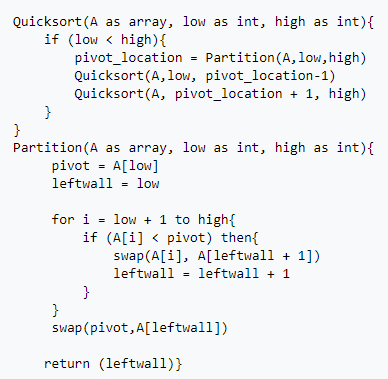Note that in the code, I took a little different approach from what I explained. Which is making the pivot the last element, by swapping it with `pointer2`, then going from `pointer1` to `pointer2`, where `pointer2` now is at the pivot at first. Sorry if it isn’t the same way I explainde it.

Code:

``````local function quicksort(array, left, right) --array is the array to sort, left is from where to start sorting and right is from where to end.
if right-left < 1 then --if it's a 1-element table, just stop
return array
end

local pointer1 = left
local pointer2 = right
local pivot = math.random(left, right) --randomly chosen pivot

array[pivot], array[pointer2] = array[pointer2], array[pivot] --swap the second pointer with the pivot

for i = pointer1, pointer2 do --go from pointer1 to pointer2
if array[i] < array[pointer2] then --each time pointer1 points at something less than pointer2
array[pointer1], array[i] = array[i], array[pointer1] --swap them , basically making pointer1 move forward

pointer1 = pointer1 + 1
end
end

array[pointer1], array[pointer2] = array[pointer2], array[pointer1] --at the end swap pointer1 and pointer2

quicksort(array, 1, pointer1-1) --apply the algorithm to both of the other parts
quicksort(array, pointer1+1, pointer2) --the part before pointer1, and the part after pointer1

return array
end

local unsortedArray = {2,5,6,9,8,5,3,4}

print(unpack(quicksort(unsortedArray, 1, 8))) --prints it ordered
``````
14 Likes

Interesting challenge. I have done this before. The only bad thing about this sort is that it cannot handle small arrays well and works best when handling larger ones.

1 Like

I’d argue the opposite actually, and since how well it handles different sized arrays are relative, I will be comparing it to another divide and conquer algorithm, MergeSort, both having the asymptotic minimum for comparison based sorting algs.

# Large Arrays

Looking at quicksort, it most commonly sorts by partitioning the original array into 2 smaller sub-arrays and recursively sorts it, while MergeSort splits it into n subarrays of length 1 before recombining everything.

Due to the way each works, MergeSort has a guaranteed time complexity of O(n log n) which you can find the proof here. However, Quicksort has a arguably worse time complexity of O(n²) due to the possibility of it partitioning the array into sub arrays of sizes `0` and `size-2`(because the partitioning index itself takes up 1 space), essentially becoming `selection sort`

This would be that as the array gets larger indefinitely, quicksort will always scale slightly worse than Mergesort, making it less than ideal for larger arrays, nullifying its advantages over mergesort.

# Smaller Arrays

What about smaller arrays? well, Quicksort easily outperforms Mergesort for relatively small arrays, due to a feel features in its implementation.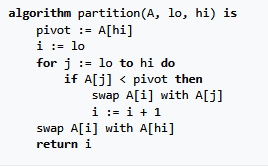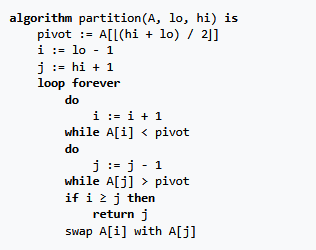(lumuto and hoare partitioning shemes, https://en.wikipedia.org/wiki/Quicksort et al)

Quicksort, as made evident by the examples above, sorts in place, and uses no auxiliary arrays, unlike mergesort, which uses many auxiliary arrays in its implementation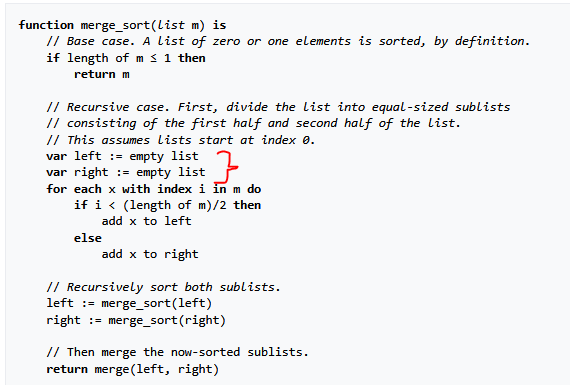(pseudocode impl. of mergesort, https://en.wikipedia.org/wiki/Merge_sort)

this , as well as having less instructions in general allows it to have a much quicker running time for smaller arrays, much like how for small arrays.

3 Likes

It might also be good to mention that, calling quicksort with an already ordered table is expensive as well.

3 Likes

that would depend on where you choose the partition, if you choose one on either end, its gonna be quadratic time, but if you choose in the middle, its linear-logarithmic time. Generally, myself and probably a lot of others would choose to use a random index as a partition.

1 Like

I see, also I think that in a normal disordered table, choosing the pivot to be the last or first element is more efficient.

1 Like

I was going to solve the problem but instead created a custom ascending and descending only sort so this is something different from the right solution.

I incorporated `math.min` and `math.max` into my sorting algorithm.

My function takes 2 parameters

Sort algorithm
``````function quicksort(array,sortAscending)

-- Prevent invalid parameters passed

assert(type(array)=="table" or array, "\r\r error : passed value must be a table \r")
assert(sortAscending == true or sortAscending == false , "\r\r sortAscending should be true or false \r")

--  local tagged = {}
--
--   for i = 1, #array do
--       if array[i] == array[#array] -- prevent 1st element == last element
--          then local tag = array[i]
--               if not array[i+1] == tag then
--               array[i] = array[i+1]
--               array[i+1] = tag
--           end
--
--   else     if array[i] == array[i+1]
--            then local tag = array[i]
--          if array[i+2] ~= tag then
--    array[i+2] = tag
--    array[i] = array[i+2]
--   end
-- end
--end
--
--   end

local temp  = {}
local sto = {}

local count = #array

local s = {}

local function getLeast(a)

if sortAscending == true then
return math.min(table.unpack(a))
else
return math.max(table.unpack(a))
end
end

local i = 0

repeat

i = i + 1

--   if i == #array then i = #array - 1
-- else
--   end

local min = getLeast(array)
sto[i] = min
--print(i.." "..min)

local indices = {}
for k, v in pairs (array) do
if v == sto[i]
-- if table.find(sto,v)
then -- table.insert(indices,k)
indices[i] = k
else
end
end

--for _,x in ipairs(indices) do
--  table.remove(array,x)
--  end
table.remove(array,indices[i])

until #sto == count

return table.unpack(sto) end

``````

Usage :

``````    Array = {9,2,2,9,2,9}
print(quicksort(Array,true))

-- true means ascending, as shown above
``````

Output :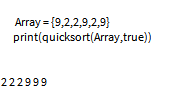### @starmaq

#### I changed my code, now it works with any numerical array with the previous limitations removed!

Works through a module:

1 Like

Thank you for taking time to make something different buts still satisfying! It’s ok if it isn’t so relevant to the challenge, as long as it’s a positive contribution.

I see. It is interesting to see that QuickSort, although can be quick at times, is not really consistent in time complexity, but MergeSort is.